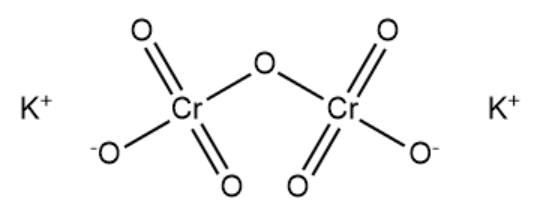Courses
Courses for Kids
Free study material
Offline Centres
MoreLast updated date: 05th Dec 2023
Total views: 280.2k
Views today: 5.80k

# Which of the following is incorrect for ${K_2}C{r_2}{O_7}$?(A) Six $(Cr - O)$ bonds are equivalent.(B) $Cr - O - Cr$ bond angle is ${126^ \circ }$ nearly.(C) Orange color is due to $d - d$ transition.(D) Oxygen is arranged tetrahedrally around chromium.Verified
280.2k+ views
Hint: ${K_2}C{r_2}{O_7}$ is an inorganic chemical reagent with the chemical name potassium dichromate. It is an odorless crystalline ionic solid with a bright red-orange color. It acts as a strong oxidizing agent. It is soluble in water but insoluble in acetone. It is highly corrosive in nature.

Complete answer: Potassium dichromate molecule has two ionic bonds between two positively charged potassium cations and the dichromate anion. The dichromate ion has two chromium atoms which are bonded to four different oxygen atoms. Two of the $Cr - O$ bonds are double bonds. One of the oxygen atoms is bonded to both chromium atoms in the molecule. The structure of potassium dichromate is given below:There are six normal $Cr - O$ bonds and there are two bridged $Cr - O$ bonds in potassium dichromate. The six normal $Cr - O$ are equivalent and different from bridged $Cr - O$ bonds. The chromate ion has tetrahedral shape and the dichromate ion has two tetrahedral sharing at one corner, with $Cr - O - Cr$bond angle equal to ${126^ \circ }$ . The color of potassium dichromate is due to ligand to metal charge transfer. Oxygen is arranged tetrahedrally around chromium in potassium dichromate.
Therefore, option C is the correct answer.

Note:
Potassium dichromate is used in cement to retard the setting of the mixture and also to improve density along with texture. It is used to clean glassware. It is also used as a medicine as an antiseptic, astringent and caustic. It is also used in photographic screen printing. It is considered as a human carcinogen as it increases the risk of developing lung cancer.